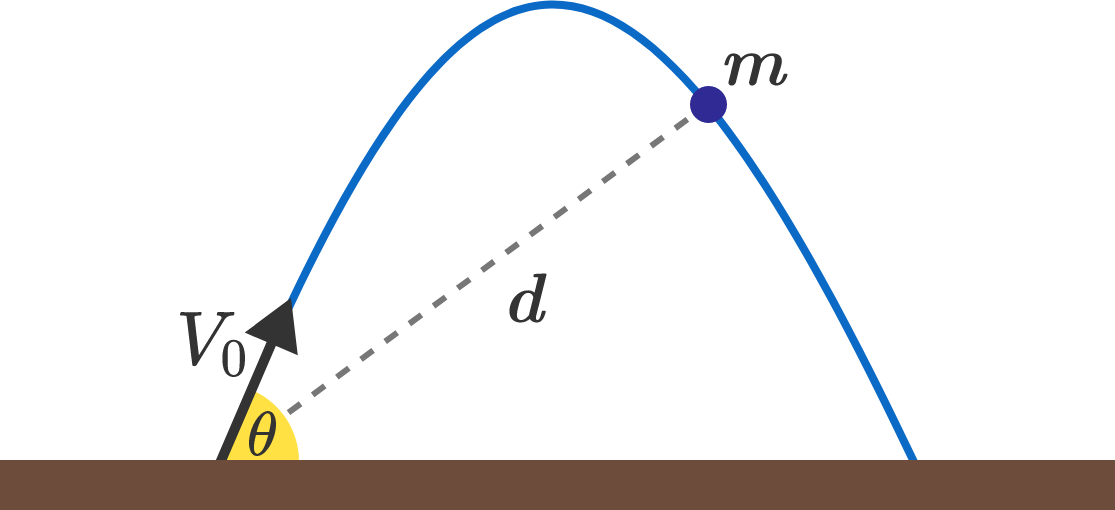# Up, Up, and Strictly Away

A ball with mass $m$ is thrown from the origin at speed $V_{0}$ toward the right on an exotic planet where the strength of gravity is $g^\prime = \frac{g}{10} = \SI[per-mode=symbol]{1}{\meter\per\second\squared}.$Let $\alpha$ be the largest possible angle such that, for all $\theta<\alpha$, the distance between the ball and its launch point will be strictly increasing for $t>0$.

What is $\tan^{2} \alpha,$ to two decimal places?


Details and Assumptions:

• $V_{0}=100 \text{ m/s}.$
• $m=1 \text{ kg}.$

Bonus: Generalize this angle for arbitrary values of $V_{0}$, $m$, and $g^\prime$.

×

Problem Loading...

Note Loading...

Set Loading...